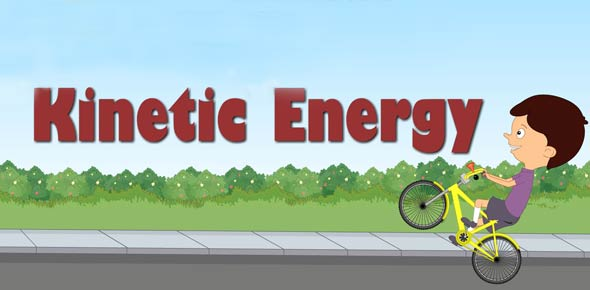# Potential & Kinetic Energy

10 Questions | Total Attempts: 800SettingsCreate your own QuizDifference between potential and kinetic energy.

• 1.
Which of the following has the most potential energy?
• A.

A car at the top of a hill

• B.

A car speeding down the hill

• C.

A person at the top of a hill

• 2.
Kinetic and potential energy both relate to:
• A.

Heat

• B.

Light

• C.

Motion

• 3.
What is potential energy?
• A.

The energy an object has due to its motion

• B.

The stored energy of an object due to its position or condition

• C.

The energy an object might have if it existed

• 4.
What is kinetic energy?
• A.

The energy an object has due to its motion

• B.

The stored energy of an object due to its position or condition

• C.

The energy an object might have if it existed

• 5.
What is an example of kinetic energy at work?
• A.

A ball lodged in a tree

• B.

A frisbee flying through the air

• C.

A car in the garage

• 6.
What does kinetic energy depend on?
• A.

The weight and volume of an object

• B.

The buoyancy of an object

• C.

The mass and speed of an object

• 7.
What would have the greatest kinetic energy?
• A.

A car driving down a hill

• B.

A person running down a hill

• C.

A ball rolling down a hill

• 8.
What has the least kinetic energy?
• A.

A car

• B.

A satellite orbiting in space

• C.

An object that is not moving

• 9.
When is kinetic energy transferred from one object to another?
• A.

When those objects pass one another

• B.

When those objects are in two places

• C.

When those objects collide

• 10.
Can you create or destroy energy?
• A.

Yes

• B.

No

• C.

Only if you are really strong

Related TopicsBack to top
×

Wait!
Here's an interesting quiz for you.